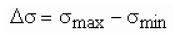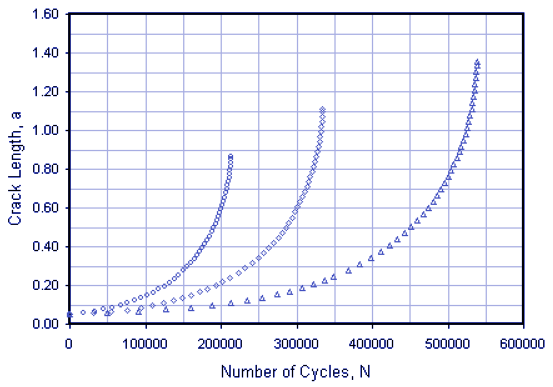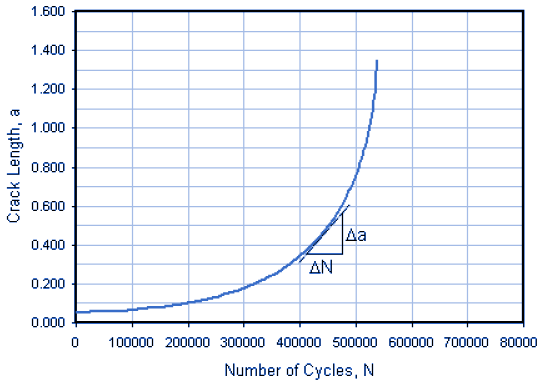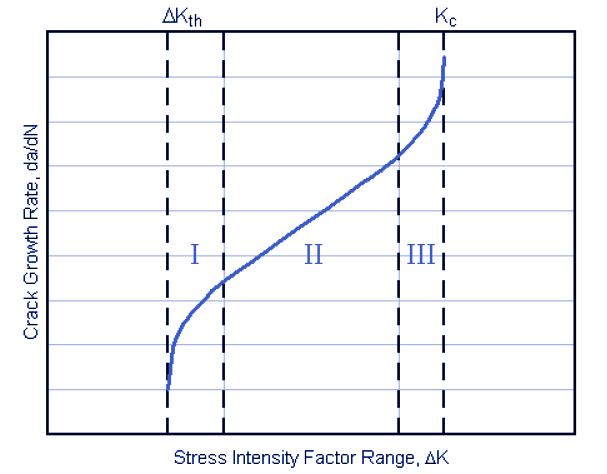Related Resources: material science

### Fatigue Crack Growth Analysis Review

Fatigue crack growth rate and the cycles-to-failure assuming a Safe-Life design approach.
The majority of the fatigue life may be taken up in the propagation of a crack. By the use of fracture mechanics principles it is possible to predict the number of cycles spent growing a crack to some specified length or to final failure. The Safe-Life (or Fail-Safe) design approach is a common design approach to predict fatigue life in structures. In this method, a component is designed such that if a crack forms, it will not grow to a critical size between specified inspection intervals. Thus, by knowing the material growth rate characteristics and with regular inspections, a cracked component may be kept in service for an extended useful life.

Constant Amplitude Crack Growth Data:

To obtain a fatigue crack growth curve, it is necessary to establish reliable fatigue crack growth rate data. Typically, a cracked test specimen is subjected to a constant amplitude cyclic load with a remote stress range given by:Typical constant amplitude crack growth data are shown in Figure 1. The crack length a is plotted versus the corresponding number of cycles N at which the crack was measured. As shown in the figure, a majority of the life of the component is spent while the crack length is relatively small.Figure 1. Constant amplitude crack growth data

Crack growth rate is defined as crack extension per cycle, da/dN. The crack growth rate is obtained by taking the slope of the crack growth curve at the crack length, a, as shown in Figure 2. Various crack growth rate curves can be generated by varying the magnitude of the cyclic loading and/or the size of the initial crack.Figure 2. Fatigue crack growth rate, da/dN

Crack Growth and the Stress Intensity Factor

Consider a crack that is propagating in the presence of a constant amplitude cyclic stress intensity factor ∆K. A cyclic plastic zone forms at the crack tip, and the growing crack leaves behind a plastic wake. If the plastic zone is sufficiently small that it is embedded within an elastic singularity zone, the stress intensity factor may still give a good indication of the stress environment at the crack tip. If two different cracks have the same stress environment (i.e., the same stress intensity factor), they behave in the same manner and show equal rates of growth. Under such conditions the rate of fatigue crack growth per cycle, da/dN, is governed by the stress intensity factor range:A typical plot of log da/dN versus log ∆K is shown in Figure 3. This curve may be divided into three regions, which are discussed in the following sections.Figure 3. Three regions of fatigue crack growth rate

Region I

At low ∆K values, crack propagation is extremely slow. Conceivably there is a threshold stress intensity value ∆Kth below which there is no fatigue crack growth or the growth is too small to measure. Although experimental verification of the existence of this threshold is difficult, ∆Kth is usually shown to be between 5 and 15 ksi-in1/2 for steels and between 3 and 6 ksi-in1/2 for aluminum alloys. The fatigue threshold decreases with an increasing stress ratio R, where R is given by:The fatigue threshold also depends on the frequency of loading and environmental conditions. Due to the sensitivity of the fatigue threshold to the environment and load history, the best method to determine the threshold value is through testing under conditions that simulate actual service conditions. Designing a component such that the ∆K for the service conditions would be below the fatigue threshold is desirable. Although this would ensure a low probability of fatigue failure, this is often impractical for design conditions due to either the low level of operating stress or the small crack size required. The threshold value may be useful when a component is subjected to low stress levels and a very large number of cycles. An example would be power trains that operate at very high speeds.

Region II

In the mid-region of stress intensities, the log da/dN versus log ∆K curve is essentially linear with the slope roughly ranging from 10-6 inch/cycle to 10-3 inch/cycle. Most structures operate in this region. Most of the current applications of linear elastic fracture mechanics (LEFM) concepts used to describe fatigue crack growth behavior are associated with Region II.

Region II is generally the largest region of the fatigue crack growth rate curve and many curve fits for this region have been suggested. The Paris and Erdogan formulation, which is commonly referred to as the Paris Equation or:The material constants C and n can be found in literature or by performing tests (ASTM E647 sets guidelines for these tests). Values of the exponent n can range from 2.0 to 7.0 with most values being between 3.0 and 4.0.

Since the stress intensity factor uses a correction factor,, that is usually a function of the crack length, the Paris Equation must often be integrated numerically. This calculation assumesis a constant determined with the crack length equal to the initial crack length, ai. Thus, the Paris Equation can be solved in closed form. It is important to note that the fatigue-life estimation is strongly dependent on ai, and is generally not sensitive to the final crack length, af (when ai << af). Large changes in af result in small changes in the calculated fatigue life. Therefore, using a constantvalue does not significantly affect the accuracy of the solution. The Stress Intensity Factor module can be used to calculate values of.
Region III

At high stress intensities, crack growth rates are extremely high and little fatigue life is involved. Region III is characterized by rapid, unstable crack growth. The crack growth rate accelerates as the maximum stress intensity factor approaches the fracture toughness of the material. In many practical engineering situations this region may be ignored because it does not affect the total crack propagation life. The point of transition from Region II and Region III behavior is dependent on the yield strength of the material, stress intensity factor, and stress ratio.

Forman's equation was developed to model Region III behavior and predicts the sharp upturn in the da/dN versus ∆K curve as the fracture toughness is approached. The Forman equation can be written as:Forman's equation is more often used to model mean stress effects in Region II, which will be discussed in the next section.

Region III is of most interest when the crack propagation life is on the order of 1000 cycles or less. However, at high stress intensities, the effects of plasticity start to influence the crack growth rate because the plastic zone size become large compared to the dimensions of the crack. Thus the problem should be analyzed by an elastic-plastic fracture mechanics approach.